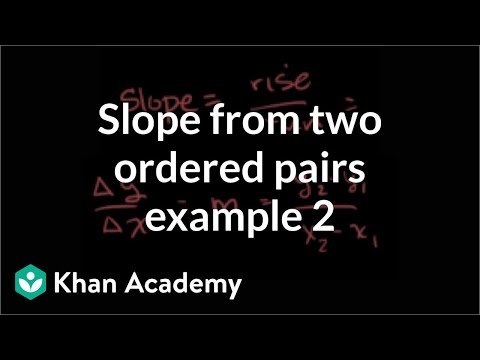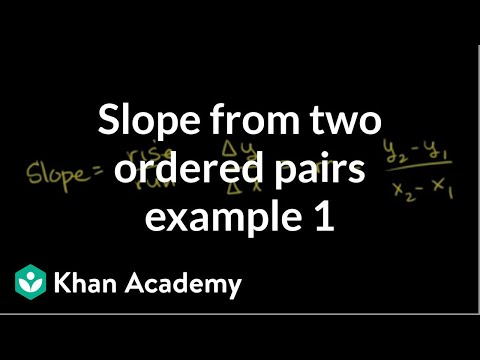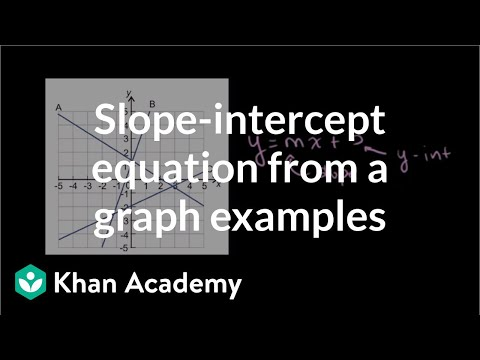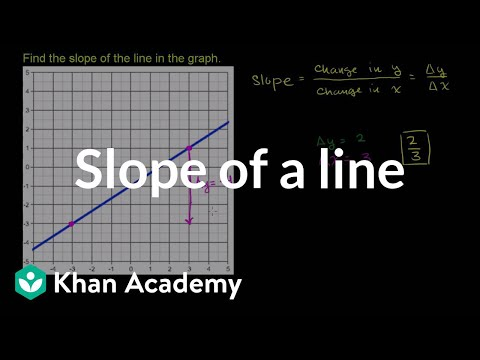# Find the Equation of a Line Using Point-Slope Form

##By PatrickJMT

Find the Equation of a Line Using Point-Slope Form - I show one complete example of finding the equation of a line using point-slope form. For more free math videos, visit http://PatrickJMT.com# Slope - Zero Versus Undefined

##By TheDouceHouse

Explain what zero slopes and undefined slopes are as well as the difference in zero slopes and undefined slopes.# Finding Slope (Part 3)

##By Khan Academy

This video lecture series on Algebra from Khan Academy includes Linear Equations, Solving Inequalities, Slope and Y-intercept, Equation of a Line, Averages, Integer sums, Taking Percentages, Systems of Equations, Ratios, Multiplying Expressions, Solving a quadratic by factoring, Imaginary Numbers, Complex Numbers, Quadratic Equations, Quadratic Inequalities, Functions, Conic sections, Circles, Ellipses, Hyperbolas.....# Equation of a Line With an Undefined Slope

##By Stephen Day

Understand the relationship between dividing by 0 and undefined slopes.# Slope from two solutions

##By Khan Academy

Another example of finding the slope between two points# Slope and Proportional Relationships

##By ElaineandTomDorlis

13 min video shows how to graph from a table and/or using an equation. Also discussed various types of slopes.# DEPRECATED Point-slope form

##By Khan Academy

DEPRECATED Point-slope form# Slope from two solutions

##By Khan Academy

An example of finding the slope between two points# Slope-intercept equation from slope and point | Algebra I | Khan Academy

##By Khan Academy

Equation of a line# Slope from two solutions

##By Khan Academy

An example of finding the slope between two points# Derivative as slope of a tangent line | Taking derivatives | Differential Calculus

##By Khan Academy

Calculus-Derivative: Understanding that the derivative is just the slope of a curve at a point (or the slope of the tangent line). All Khan Academy content is available for free at www.khanacademy.org# Algebra: Slope and Y-intercept intuition

##By Khan Academy

This video lecture series on Algebra from Khan Academy includes Linear Equations, Solving Inequalities, Slope and Y-intercept, Equation of a Line, Averages, Integer sums, Taking Percentages, Systems of Equations, Ratios, Multiplying Expressions, Solving a quadratic by factoring, Imaginary Numbers, Complex Numbers, Quadratic Equations, Quadratic Inequalities, Functions, Conic sections, Circles, Ellipses, Hyperbolas.....# The slope of a linear function

##By MathPlanetVideos

Find the slope# Slope from two solutions

##By Khan Academy

Another example of finding the slope between two points# Khan Academy - Finding slope and y intercept

##By Khan Academy

This video lecture series on Worked Examples in Algebra from Khan Academy includes Solving Equations, Solving Word Problems, Solving for a variable, Absolute Value and Number Lines, Patterns in Sequences, Functional Relationships, Domain and Range, Rate Problems, Linear Functions, Slope of a Line, X and Y intercepts, Equation of a Line, Parallel Lines, Perpendicular Lines, Solving Inequalities and more# Graph from slope-intercept equation example | Algebra I

##By Khan Academy

This video lecture series on Worked Examples in Algebra from Khan Academy includes Solving Equations, Solving Word Problems, Solving for a variable, Absolute Value and Number Lines, Patterns in Sequences, Functional Relationships, Domain and Range, Rate Problems, Linear Functions, Slope of a Line, X and Y intercepts, Equation of a Line, Parallel Lines, Perpendicular Lines, Solving Inequalities and more..# Finding the slope of a line from its graph | Algebra I

##By Khan Academy

This video lecture series on Worked Examples in Algebra from Khan Academy includes Solving Equations, Solving Word Problems, Solving for a variable, Absolute Value and Number Lines, Patterns in Sequences, Functional Relationships, Domain and Range, Rate Problems, Linear Functions, Slope of a Line, X and Y intercepts, Equation of a Line, Parallel Lines, Perpendicular Lines, Solving Inequalities and more...# Graphing Lines in Slope-Intercept form y=mx+b

##By ProfRobBob

I introduce how to graph a line in slope intercept form. I also discuss graphing vertical and horizontal lines.# Slope-intercept equation from a graph

##By Khan Academy

Slope-intercept equation from a graph# Slope from a graph

##By Khan Academy

Slope of a line